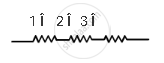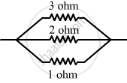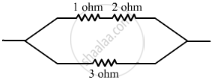Share
Notifications

View all notifications
Books Shortlist
Your shortlist is empty

# You Are Given Three Resistances of 1, 2 and 3 Ohms. Shows by Diagrams, How with the Help of These Resistances You Can Get: (I) 6 ω (Ii) 6/11 ω (Iii) 1.5 ω - CBSE Class 10 - Science

Login
Create free account

Forgot password?
ConceptResistance of a System of Resistors - Resistors in Parallel

#### Question

You are given three resistances of 1, 2 and 3 ohms. Shows by diagrams, how with the help of these resistances you can get:

(i) 6 Ω
(ii) 6/1 Ω
(iii) 1.5 Ω

#### Solution

To get a value of 6 Ω, all the resistors are to be connected in series as shown below:Net resistance, R = R1 + R2 + R3
R = 1 Î + 2 Î + 3 Î = 6 Î

To get a value of 6 / 11 Ω, all the resistors are to be connected in parallel as shown below:When tree resistance is conencted in parallel their resultant is given by

1/R=1/R_1+1/R_2+1/R_3

R1=3 Ω

R_2=2Ω

R_3=1 Ω

1/R=1/3+1/2+1/1

1/R=(2+3+6)/6

1/R=11/6

R=6/11Ω

To get a value of 1.5 Ω, the 1 Ω and 2 Ω resistors should be connected in series and this arrangement should be connected in parallel with the 3 Ω resistor as shown below:The resistors of resistance 1 Î and 2 Î are in series. Therefore, their net resistance is:

R=R_1+R_2

R=1I+2I

R=3I

This 3 Î is connected in parallel with another 3 Î resistance.
Therefore, net resistance will be:

1/R=1/R_1+1/R_1

1/R=1/3+1/3

1/R=2/3

R=3/2=1.5Ω

1R=1R_1+1R_2  1R=13+131R=23R=32=1.5Ω

Is there an error in this question or solution?

#### Video TutorialsVIEW ALL 

Solution You Are Given Three Resistances of 1, 2 and 3 Ohms. Shows by Diagrams, How with the Help of These Resistances You Can Get: (I) 6 ω (Ii) 6/11` ω (Iii) 1.5 ω Concept: Resistance of a System of Resistors - Resistors in Parallel.
S